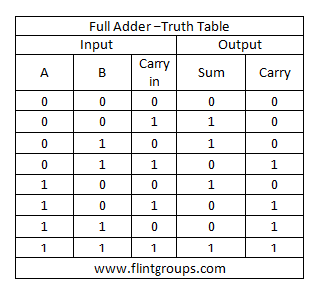# What is meant by Arithmetic Circuits?

## Arithmetic Circuits :

Arithmetic Circuits are nothing but the logic circuits,which is used to Complete some Arithmetic Functions like addition,subtraction,multiplication,division,parity calculation etc.Arithmetic Logic Circuits are always a combinational logic circuits because the output is totally depends on the current input not depends on the previous inputs and outputs.

ADDERS : Adders are the basics of all hose Arithmetic Circuits.Name itself explain it nature it is used to add two binary number and provides sum and carry as a output.basically we have two types of adders.

HALF ADDER : A Circuit which is used to add two single bit binary numbers is called half adder.suppose if we add two binary numbers each number is high state that mean Value of both the number is 1.then carry formed at its output.The Truth Table of the Half Adder is given below.From the Truth Table we found the logic expression for the outputs of aum and carry.

Derivation Equation for sum.in the above truth table the value of sum is 1 in only two combinations of inputs.in the first combination the value of A is 0 so it is considered as A' The value of B is 1 so it is taken as B.Then this combination is A'B.Like in such a Way in Second Combination Value of A is 1 so it is A The Value of B is 0 so it is considered as B' then the equation for this combination is AB'.
Totally the Equation of Sum is Expressed as

Sum=A'B+AB' =AB

Like That you can find for Carry The we got the equation of carry as

Carry = AB.

Logic Diagram Of Half Adder is Given Below the Ex-OR Gate is used to produce the sum and an AND Gate is used to produce the carry.FULL ADDER : A logic Circuit Which is used for adding Three Single bit Binary numbers is known as Full Adder.The Truth Table of Full Adder is Shown Below.Like Half Adder the expression for Sum here is .

Sum = A'B'C+A'BC'+AB'C'+ABC.
By simplifying This
=A'(B'C+BC')+A(B'C'+BC).
The Value of B'C'+BC can be reduced as
B'C'+BC=((B'C'+BC)')'
=((B'C')').(BC)')'
=((B''+C').(B'+C'))'
=((B+C).(B'+C'))'
=(BB'+BC'+B'C+CC')'
=(BC'+B'C)'  Because BB'=CC'=0
Sustitute the Value of  B'C'+BC in A'(B'C+BC')+A(B'C'+BC).

Then
Sum=A'(B'C+BC')+A(BC'+B'C) '
=A'(BC)+A(BC)'
Sum=ABC

If you feel This Steps are too hard the go for K-MAP you are getting results in single step.

Similarly The Expression for Carry = A'BC+AB'C+ABC'+ABC.
After Simplification we got
Carry = AB+BC+CA.

Other Wise we may Written as
Carry =(AB)C+AB

The Logic Diagram of the Full Adder is Given Below.1.2.